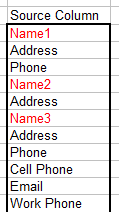Variable Block Length Column To Table

This page describes code for spliting apart a column of data into a table when the blocks in the source column have a variable number of elements.Introduction

On the Column To Table page we looked at formulas that can be used to create a two-dimensional table from a column of data. This works fine as long as each block of data in the column has a fixed number of elements. However, it is often the case that the blocks of data in the source column are variable length. Some blocks may have 3 elements while other blocks have 5 elements. The code here will create a two dimensional table each column of which is one block of value from the source column data.

For the purposes at hand, we will assume that there is some type of delimiting value at the beginning of each data block in the source column that indicates where one block begins following the elements of the previous block. This may be a constant string, an empty cell, or a string that can be evaluated with the Like operator. There may be any number of cells (including empty cells) between two delimited cells. It is possible also that two delimiter values occur in adjacent cells with no data between them. A sample source column is shown below.In this image, the delimiter cells are colored in red. This is for illustration only. In practice, any cell formatting is irrelevant and is ignored (and thus the cell formatting will not appear in the result table. The delimiter cells contain the text NameN, where N is some arbitrary number. Because the code uses the Like operator, all of these values will be recognized as a block delimiter. The delimiter would be specified as NAME*, where the * indicates a match of zero or more characters. See the VBA help file for the Like operator for a full discussion of available formats used with Like.

If the delimiter is not a blank cell, each delimiter is included in the first row of the results table. If the delimiter is a blank cell, these blank cells do not appear in the results table.

The code for the VarColToTable procedure which creates the two dimensional table from the source column is shown below.  You will need to change the values annotated by <<< to match the requirements of your workbook and data.

```Sub VarColToTable()
Dim R As Range
Dim Dest As Range
Dim DR As Range
Dim LastRow As Long
Dim SourceWS As Worksheet
Dim DestinationWS As Worksheet

' <<< Change "NAME*" to the cell text that indicates
' the start of a new block. The code uses LIKE so
' wildcards are allowed.
Const C_DELIMITER = "NAME*"
' <<< Change "E1" to the first cell on DestinationWS
' where the blocked data is to begin.
Const C_DEST_FIRST_CELL = "E1"
' <<< Change "Sheet1" to the worksheet that contains
' the original data.
Set SourceWS = Worksheets("Sheet1")
' <<< Change "Sheet2" to the worksheet to which the
' bloced data should be written.
Set DestinationWS = Worksheets("Sheet1") '<<< CHANGE
' <<< Change "A1" to the first cell of the original data.
Set R = SourceWS.Range("A1")

With SourceWS
' get the last used row in the column specified by R.
LastRow = .Cells(.Rows.Count, R.Column).End(xlUp).Row
End With

If Not UCase(R) Like UCase(C_DELIMITER) Then
MsgBox "The first item in the list must conform to C_DELIMITER '" + C_DELIMITER & "'."
Exit Sub
End If

Do Until R.Row > LastRow
' loop until we go down past LastRow.
If UCase(R.Text) Like C_DELIMITER Then
' R points to a delimeter cell value
If Dest Is Nothing Then
' if this is the first block, Dest will be
' Nothing, so set it to the first destination cell.
Set Dest = DestinationWS.Range(C_DEST_FIRST_CELL)
If C_DELIMITER = vbNullString Then
' if blank cells are used a the delimiter, adjust
' Dest up one row so the empty cells do not appear
' in the split data table.
Set Dest = Dest(0, 1)
End If
Else
' this is not the first block. Move Dest to point to
' the next column to the right.
Set Dest = Dest(1, 2)
End If
' Set DR, which points to where elements in a block
' to the Dest cell to start a new output block location.
Set DR = Dest
End If
' Put the value of R in DR.
DR.Value = R.Text
' Move DR down one row so the next element will be below it.
Set DR = DR(2, 1)
' Move R down one row in the original data.
Set R = R(2, 1)
Loop
End Sub
```

The following show the resulting table created by the code:This page last updated: 18-December-2008.

-->